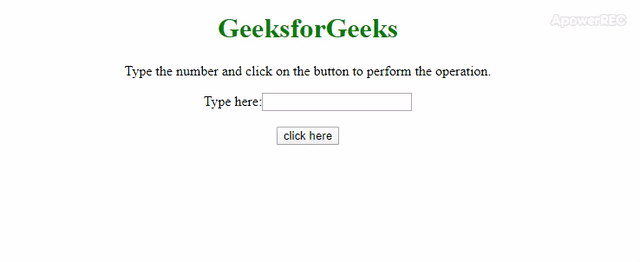# Split a number into individual digits using JavaScript

In this article, we will get some input from user by using <input> element and the task is to split the given number into the individual digits with the help of JavaScript. There two approaches that are discussed below:

Approach 1: First take the element from input element in string format (No need to convert it to Number) and declare an empty array(var res). Visit every character of the string in a loop on the length of the string and push the character in the array(res) by using push() method.

• Example: This example implements the above approach.

 `  ` `<``html``>  ` ` `  `<``head``>  ` `    ``<``title``>  ` `        ``Split a number into individual ` `        ``digits using JavaScript ` `    ``  ` `     `  `    ``<``style``> ` `        ``body { ` `            ``text-align: center; ` `        ``} ` `             `  `        ``h1 { ` `            ``color: green; ` `        ``} ` `        ``#geeks { ` `            ``color: green;  ` `            ``font-size: 29px; ` `            ``font-weight: bold; ` `        ``} ` `    `` ` `  ` ` `  `<``body``>  ` `    ``<``h1``>GeeksforGeeks ` `     `  `    ``<``p``> ` `        ``Type the number and click on the button  ` `        ``to perform the operation. ` `    `` ` `     `  `    ``Type here:<``input` `id` `= ``"input"` `/> ` `         `  `    ``<``br``><``br``> ` `     `  `    ``<``button` `onclick` `= ``"GFG_Fun();"``> ` `        ``click here ` `    `` ` `     `  `    ``<``p` `id` `= ``"geeks"``> ` `     `  `    ``<``script``> ` `        ``var down = document.getElementById('geeks'); ` `         `  `        ``function GFG_Fun() { ` `            ``var str = document.getElementById('input').value; ` `            ``res = []; ` `             `  `            ``for (var i = 0, len = str.length; i < ``len``; i += 1) { ` `                ``res.push(+str.charAt(i)); ` `            ``} ` `             `  `            ``down.innerHTML` `= ``"["` `+ res + "]"; ` `        ``} ` `    ``  ` `  ` ` `  `          `

• Output:Approach 2: First take the element from input element in string format (No need to convert it to Number) and declare an empty array(var res). Split the string by using split() method on (”) and store the spitted result in the array(str).

• Example: This example implements the above approach.

 ` ` `<``html``> ` ` `  `<``head``> ` `    ``<``title``> ` `        ``Split a number into individual digits ` `    `` ` `     `  `    ``<``style``> ` `        ``body { ` `            ``text-align: center; ` `        ``} ` `         `  `        ``h1 { ` `            ``color: green; ` `        ``} ` `         `  `        ``#geeks { ` `            ``color: green; ` `            ``font-size: 29px; ` `            ``font-weight: bold; ` `        ``} ` `    `` ` ` ` ` `  `<``body``> ` `    ``<``h1``>GeeksforGeeks ` `     `  `    ``<``p``> ` `        ``Type the number and click on the ` `        ``button to perform the operation. ` `    `` ` `     `  `    ``Type here: <``input` `id``=``"input"` `/> ` `     `  `    ``<``br``><``br``> ` `     `  `    ``<``button` `onclick``=``"GFG_Fun();"``> ` `        ``click here ` `    `` ` `     `  `    ``<``p` `id``=``"geeks"``> ` `     `  `    ``<``script``> ` `        ``var down = document.getElementById('geeks'); ` ` `  `        ``function GFG_Fun() { ` `            ``var n = document.getElementById('input').value; ` `            ``var str = n.split(''); ` `            ``down.innerHTML = "[" + str + "]"; ` `        ``} ` `    `` ` ` ` ` `  ` `

• Output:My Personal Notes arrow_drop_upCheck out this Author's contributed articles.

If you like GeeksforGeeks and would like to contribute, you can also write an article using contribute.geeksforgeeks.org or mail your article to contribute@geeksforgeeks.org. See your article appearing on the GeeksforGeeks main page and help other Geeks.

Please Improve this article if you find anything incorrect by clicking on the "Improve Article" button below.